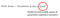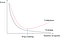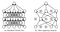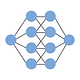# Intuitive Deep Learning Part 1b: Introduction to Neural Networks

## How do we make neural networks work? Some nitty-gritty details to take note of!

This is the second post in the introductory series of Intuitive Deep Learning, where we go into the nitty-gritty of making neural networks work! If you want more from this series, please follow the publication “Intuitive Deep Learning”.

Where we left off: In Part 1a, we started to understand what machine learning is and some high-level concepts to approaching Machine Learning:

Machine Learning specifies a template and finds the best parameters for that template instead of hard-coding the parameters ourselves. A neural network is simply a complicated ‘template’ we specify which turns out to have the flexibility to model many complicated relationships between input and output. Specifying a loss function and performing gradient descent helps find the parameters that give the best predictions for our training set.

Nevertheless, we left a few questions unanswered in the previous post, which will be the topic of this post. In part 1b, we will be going into more nitty-gritty aspects of Machine Learning, but they are all necessary and crucial components to make Machine Learning models work.

Question: If my model has the lowest loss value on my training data-set, does that mean my model is the best?

Well, no. This might come as a surprise because there is a subtle point here.

Let’s take the problem of classifying whether an X-ray image shows an anomaly or not. The aim here, as obvious as it sounds, is to build a classifier for X-ray images that will work for all X-ray images. The subtle point to note is this: A model that works well on training data does not mean the model’s performance will generalize to all X-ray images.

To give an extreme example, suppose we give the neural network exactly two images, one of an X-ray with anomaly (Image 1) and one of an X-ray without anomaly (Image 2). The model could learn something to the effect of:

1. If I see Image 1 exactly, predict that it has an anomaly with 100% probability.
2. If I see Image 2 exactly, predict that it has no anomaly with 100% probability.
3. If I don’t see either Image 1 or Image 2, just predict a 50% probability of anomaly.

Notice that the loss on the training dataset (Image 1 and Image 2) is zero, which is the lowest it can receive. After all, it predicted the correct class with 100% probability on our training set. However, we can’t expect this model to be a very good model ready to be deployed in hospitals, because the model does not generalize to other unseen X-ray images (where unseen means that the model has not trained on that image).

This is the problem of overfitting: our model has fit so extremely to the training data that it fails to generalize to other examples. Two questions naturally arise:

1. How do I tell if my model is overfitting?
2. What’s the solution for overfitting?

We start with the first question. The problem here was that training loss (loss on the training dataset) failed to give us an accurate picture of how well the model generalizes to unseen images. Thus, we often split our dataset into a training dataset and a validation dataset (often also called a dev set). As the name suggests, we train our model on the training dataset, and we validate the model’s ability to perform on unseen data in the validation set. The sign of overfitting is then a low training loss but a high dev loss, just like the over-fitting model we described above.

The model we choose is then the model that performs best on the validation set, rather than the training set (due to the overfitting problem).

Now if we know our model is overfitting, what can we do? Here are a few strategies we can adopt, which we call regularization:

Strategy 1: L2 Regularization. The problem with over-fitted models is that we often have some wild crazy model that works well only for the training examples. If we penalize wild crazy models (by penalizing extremely large parameters), we can reduce over-fitting. How can we introduce this penalty? Simple — add the penalty to the loss function! The penalty is the squared value of the parameters (scaled by some constant number), so the larger the parameters, the higher the loss will be.New loss when we add L2-regularization that penalizes overly large parameters (i.e. wild and crazy models)

Remember that the loss function helps us what the ‘best parameters’ are; we are thus effectively saying that the ‘best parameters’ are ones that both minimize the training loss and have small parameters.

Strategy 2: Early Stopping. If we plot the function of training loss over time, we expect it to constantly fall because the parameters will move in a way that reduces training loss. If we plot the function of dev loss over time, however, we see that it takes a U-shape. The intuition is that the dev loss will decrease as the model gets better on the training set (as we expect), but beyond a certain point, the model is fitting so well to the training set that it does not generalize well to the validation set.How early stopping works. Most models have a U-shaped validation loss, so we stop where the dev loss is the lowest. Image taken from: http://fouryears.eu/2017/12/06/the-mystery-of-early-stopping/

As we’ve discussed previously, we want to take the point where the dev-loss is the lowest. This means that we take the model at the bottom of the U-shape of the dev-loss curve as our final model.

Strategy 3: Dropout. Dropout works as follows: during the training of our model, we randomly cause some of the neurons to be excluded from the neural network (i.e. drop out) at each step with a fixed probability. Since the model won’t know which neurons will be dropped out at each step, it is forced to not overly rely on key neurons, thereby preventing wild and crazy models.How dropout works: we exclude some of the neurons from the neural network (as if they don’t exist) randomly during the training phase. Image taken from Srivastava, Nitish, et al. ”Dropout: a simple way to prevent neural networks from overfitting”, JMLR 2014

It seems strange at first glance, but it is a surprisingly effective strategy for dealing with overfitting.

The exact math behind these strategies are not the focus of this post; rather, it is just a flavor for how we might deal with this overfitting problem.

Summary: Overfitting occurs when our model has fitted so well to the training dataset that it has failed to generalize to unseen examples. This is characterized by a high dev loss and a low train loss, and can be addressed with regularization techniques.

Question: There are so many ‘neural network templates’ that we can specify. How do we know what is the best ‘neural network template’ to use?

It is true that there are many different type of neural networks we can specify. We get a different template by simply varying the number of hidden layers, the number of neurons in each hidden layer, the type of activation functions and so on. These are called hyper-parameters because they define the template (whereas the parameters are the numbers inside the template).

As mentioned above, we take the model of choice to be the model that performs best on the validation set. We simply need to search for hyper-parameters that perform the best on the validation set. The Machine Learning pipeline looks a little bit like this:

1. Specify some hyper-parameters (the template)
2. Train on the training dataset (filling in the parameters)
3. Record the validation loss
4. Repeat Steps 1 to 3 with a different set of hyper-parameters (many times)
5. Pick the model with the lowest validation loss as our best model
6. Run the chosen model on a separate test dataset (see Footnote 1)

If you recall what Machine Learning is about (in Part 1a), we need to not only find the best numbers to fill in the template (parameters) but we also need to find the best template (hyper-parameters), as we do in Step 4. Right now, the field of Machine Learning remains a highly empirical one; we experiment with different hyper-parameters and see what works without always fully knowing why it works so well with specific numbers. Hopefully, the theory behind why it works so well will soon catch up with the empirical breakthroughs we’ve seen.

A small caveat: It is misleading to say that all our hyper-parameters relate to the model architecture. The truth is, there are other hyper-parameters we might wish to try, such as the learning rate in our gradient descent algorithm. These are things that will change the model’s performance, but the scope is outside this post.

Summary: The model we choose is the one that performed the best on our validation set. We can experiment with different hyper-parameters to specify different templates that can give us a lower validation loss.

Question: Gradient descent iteratively takes steps towards the point where it has the lowest loss. How do we decide where our starting point would be to take our first step?

This is a question on initialization. The most important thing is to not initialize our parameters to be the same for all parameters (such as all starting from zero). The problem with doing so is that if our parameters are the same from the start, then the neurons in the hidden layer would give the same output (i.e. zero).

If all the neurons in the hidden layer gives the same output (because they are the same), the update that they get from gradient descent will be the same too! After all, why should the update be any different if the neurons are exactly the same? Now if the update is the same and the starting point is the same, the neurons will never be different from each other, no matter how long you’ve trained it. In that case, you might as well have just one neuron in your hidden layer!

A simple method to break this symmetry is by having random numbers close to zero as our initialization. There are other little tricks to have a better initialization, but this will be covered in a later post.

Summary: When we do gradient descent, we want to initialize the parameters with some randomness to break the symmetry.

Question: Are there other optimization algorithms to find the best parameters to put inside our template?

Yes, of course. There are a few problems with gradient descent, one of which is that it can often be extremely slow. Recall that gradient descent calculates the direction by taking the derivative of the overall loss with respect to the parameters. The overall loss is the mean of the loss of ALL our training examples. Imagine if we had 1 million images (which is the order of magnitude in current deep-learning datasets); we’d have to calculate the loss of 1 million examples every time we want to make one gradient descent step!

What we do in practice is that we calculate the loss of a subset (batch) of training examples as a proxy for our overall loss. This subset is called the mini-batch, and this method is called the mini-batch gradient descent. Note that after each step, we do our calculations for the loss function on the next batch (if we calculate on one batch only, then there’s no point in having all those examples!)

The idea is that if we choose our batch-size to be large enough, we get a good enough proxy for the overall loss, without having to make that computationally expensive calculation on the loss function of 1 million images! If we define the batch size to be 1, this is called stochastic gradient descent. We call a complete pass through the full dataset an epoch.

There are many other more advanced methods that help us do better in our optimization step, including momentum, RMSprop, and Adam to name a few. This will be covered in later posts on more advanced topics.

Summary: There is also another method called mini-batch gradient descent, which helps us achieve faster updates as it does not calculate the loss over all training examples.

Consolidated Summary: Overfitting occurs when our model has fitted so well to the training dataset that it has failed to generalize to unseen examples. This is characterized by a high dev loss and a low train loss, and can be addressed with regularization techniques. The model we choose is the one that performed the best on our validation set. We can experiment with different hyper-parameters to specify different templates that can give us a lower validation loss. When we do gradient descent, we want to initialize the parameters with some randomness to break the symmetry. There is also another method called mini-batch gradient descent, which helps us achieve faster updates as it does not calculate the loss over all training examples.

What’s Next: So far, we’ve covered at a very high level the basic concept of Machine Learning as well as dived a little into the nitty-gritty details behind making Machine Learning models work.

In the next post, Part 2, we will apply neural networks to understanding images. It turns out that in image data (rows and columns of individual pixels), there are some innovations in our model architecture that helps us exploit important attributes of image data.

Footnotes:

1. Evaluation / Test Set

At this point, you might ask — Why do we need to split our dataset into the training dataset, the validation dataset and the test dataset? Recall that the validation dataset gives us an accurate measure of whether we have over-fitted our parameters to the training dataset.

Similarly, the test dataset gives us an accurate measure of whether we have over-fitted our hyper-parameters to the validation dataset. If we choose the best numbers to fit the hyper-parameter based on our dev set, we might realize that those hyper-parameters work well for our dev set but not for general unseen cases.

Written by

Written by

## Joseph Lee Wei En

#### I want to make Deep Learning concepts as intuitive and as easily understandable as possible by everyone! Follow my publication to learn more!